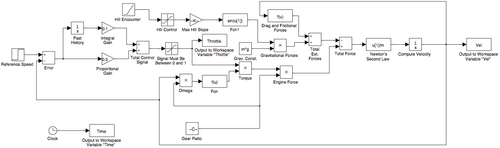# Difference between revisions of "Cruise control"

## Contents

This page documents the cruise control system that is used as a running example throughout the text. A detailed description of the dynamics of this system are presented in Chapter 3 - Examples. This page contains a description of the system, including the models and commands used to generate some of the plots in the text.

## Introduction

Cruise control is the term used to describe a control system that regulates the speed of an automobile. Cruise control was commercially introduced in 1958 as an option on the Chrysler Imperial. The basic operation of a cruise controller is to sense the speed of the vehicle, compare this speed to a desired reference, and then accelerate or decelerate the car as required. The figure to the right shows a block diagram of this feedback system.

A simple control algorithm for controlling the speed is to use a "proportional plus integral" feedback. In this algorithm, we choose the amount of gas flowing to the engine based on both the error between the current and desired speed, and the integral of that error. The plot on the right shows the results of this feedback for a step change in the desired speed and a variety of different masses for the car (which might result from having a different number of passengers or towing a trailer). Notice that independent of the mass (which varies by a factor of 3), the steady state speed of the vehicle always approaches the desired speed and achieves that speed within approximately 5 seconds. Thus the performance of the system is robust with respect to this uncertainty.

The file {{{2}}} contains a fairly complete model of a cruise controller for a car, depicted below.## MATLAB model

An alternative model for the system is a MATLAB model suitable for use with the 'ode45' function. The file {{{2}}} contains a nonlinear model of the car dynamics consistent with the description given in Section 3.1.

## Exercises

The following exercises make use of the cruise control model described here: